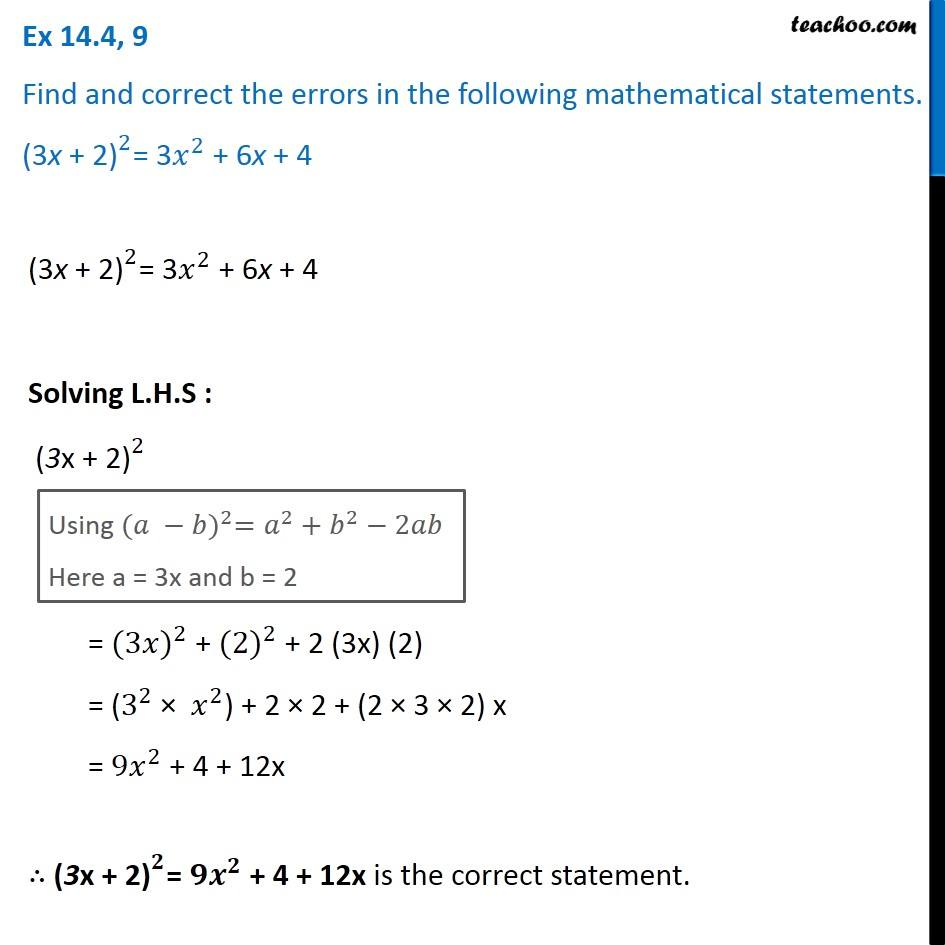Ex 14.4

Chapter 14 Class 8 Factorisation
Serial order wiseGet live Maths 1-on-1 Classs - Class 6 to 12

### Transcript

Ex 14.4, 9 Find and correct the errors in the following mathematical statements. 〖"(3x + 2)" 〗^2= 3𝑥^2 + 6x + 4 〖"(3x + 2)" 〗^2= 3𝑥^2 + 6x + 4 Solving L.H.S : 〖"(3x + 2)" 〗^2 Using 〖(𝑎 −𝑏)〗^2=𝑎^2+𝑏^2−2𝑎𝑏 Here a = 3x and b = 2 = 〖(3𝑥)〗^2 + 〖(2)〗^2 + 2 (3x) (2) = (3^2 × 𝑥^2) + 2 × 2 + (2 × 3 × 2) x = 〖9𝑥〗^2 + 4 + 12x ∴ 〖"(3x + 2)" 〗^𝟐= 〖𝟗𝒙〗^𝟐 + 4 + 12x is the correct statement.